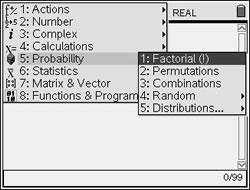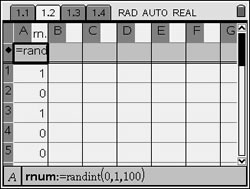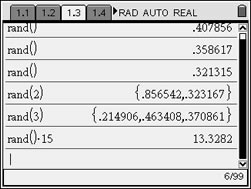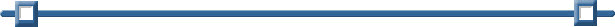Generating Random Numbers

Random numbers can be quickly and easily generated by using the graphing calculator.
Let's start our investigation by looking at generating random integers.

 Generating Random Integers on the Home Screen: (good for games)From the Calculator page, press the MENU key, #5 Probability to access #4 Random options.For integers, choose #2 Integers. Enter the smallest value needed, followed by the largest value.  Hitting ENTER will generate the random integers. (Random values may repeat.) This example generates random numbers from 1 to 25 (good for Bingo).Adding a third parameter indicates the number of random integers that will appear on the screen at one time. Keep hitting ENTER to generate values.
 Generating Random Integers in Lists: (good for statistical studies)From Lists & Spreadsheets, name the list "rnum". In the formula cell, enter = randInt(1,25,20) to generate 20 random numbers between 1 and 25 inclusive.Entering = randInt(0,1,100) could simulate 100 tosses of a fair coin. Hitting CTRL - R will recalculate the list and give you a new set of random values. Such lists can be used to simulate the toss of one, or more, fair coin(s).  The number of entries represents the number of tosses.  An even random number represents heads, while an odd number represents tails. If tossing one coin, use sum command (from Catalog) to count the number of heads, where heads are 1, and tails are 0.From Calculator page, Catalog, S, choose sum( and fill in the name of the list. There were 49 heads (or 1's) in this list.
 Using the rand command: (not integers) The rand( )command will generate random numbers, not integers, between 0 and 1. From the Random listing, choose option #1 Number. The rand( )command will create a random number between 0 and 1. No value is needed in the parentheses. If a value is placed in the parentheses, it signifies the number of random values displayed. To generate a random number between 0 and 15, enter rand( )*15.Re-Seeding the Random Number Generator:
Calculators (and computers) are not capable of creating "truly random" numbers.  They create what are called "pseudo-random" numbers, meaning they use a formula to create the values.  To engage this formula, the calculator uses a starting value, called a "seed", and then creates the random numbers based upon this seed.  If two calculators start with the same seed value, they will generate the same sequence of random values.From the Random listing, choose option #6 Seed. Enter ANY value as the seed number. If you wish, you can control the starting "seed" value. To seed the random generator, choose a seed value and store it using the RandSeed command. Now, start generating your random values. Notice that when the seed was reset to the first choice, the rand( ) command gave the same result. If you feed two calculators the same seed value, they will each produce the same result when rand( )is entered. Calculators will default to the same seed value.

If you wish to ensure that each student in the class has a different set of random numbers, assign a different number to each student as their seed value.  You could also have the students enter their birth date as the random seed (04021990), assuming no two students have the same birth date.